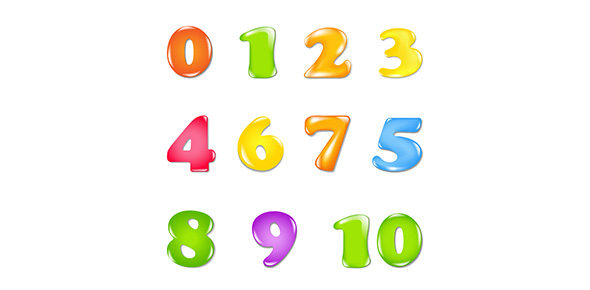# Operations With Rational Numbers

5 Questions | Attempts: 333
ShareSettingsMathematical operations with decimals and percents.

• 1.
45.17 + 327.19 =
• A.

37.92

• B.

372.96

• C.

778.9

• 2.
58.49 x 22.118 =
• A.

1293.68182

• B.

12936.8182

• C.

129.37

• 3.
457.89 - 32.74 =
• A.

13.15

• B.

425.15

• C.

490.63

• 4.
210.45 / .05 =
• A.

42.90

• B.

420.9

• C.

4209

• 5.
How could you find 15% of \$72.00
• A.

Convert 15% to the decimal .15 and multiply \$7.20 times .15.

• B.

10% of \$72.00 is \$7.20 plus 5% of \$72.00 is half of 10%, so \$7.20 plus \$3.60 equals \$10.80.

• C.

Find 1% of \$72.00 which is .72 and multiply by 15.

• D.

A

• E.

A and B

• F.

All of the above.

## Related TopicsBack to top
×

Wait!
Here's an interesting quiz for you.